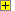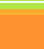Physics GCSE
Unit 1Random calculations based on formulae in AQA's GCSE Physics Unit 1.
As well as a random selection of the formula, the equation is randomly transposed.Show Calculator